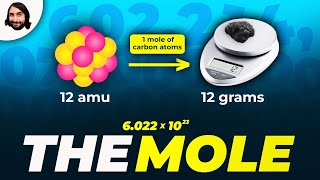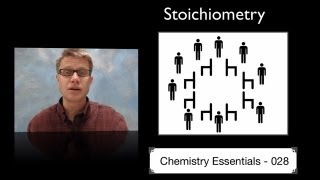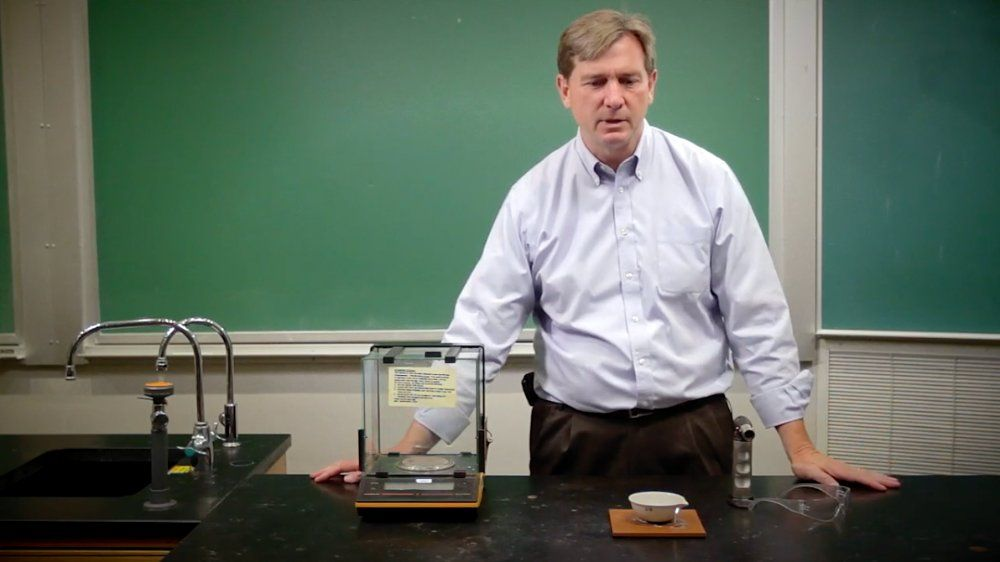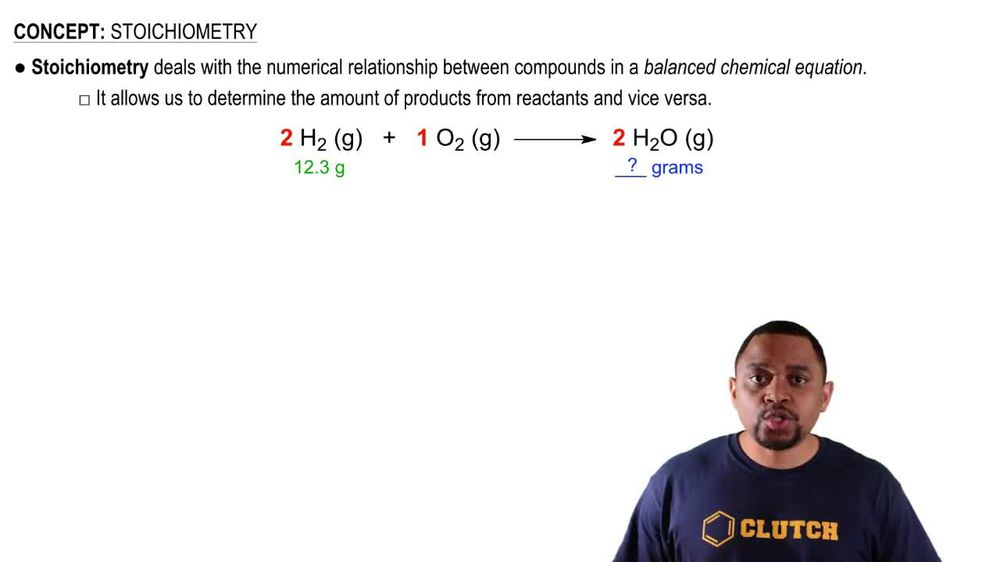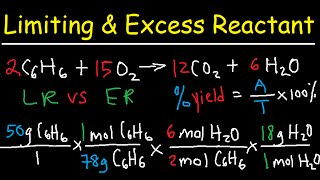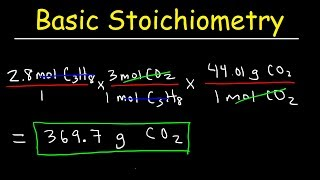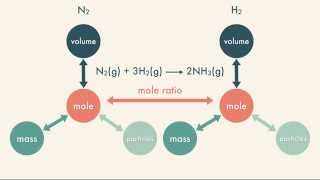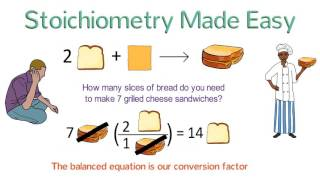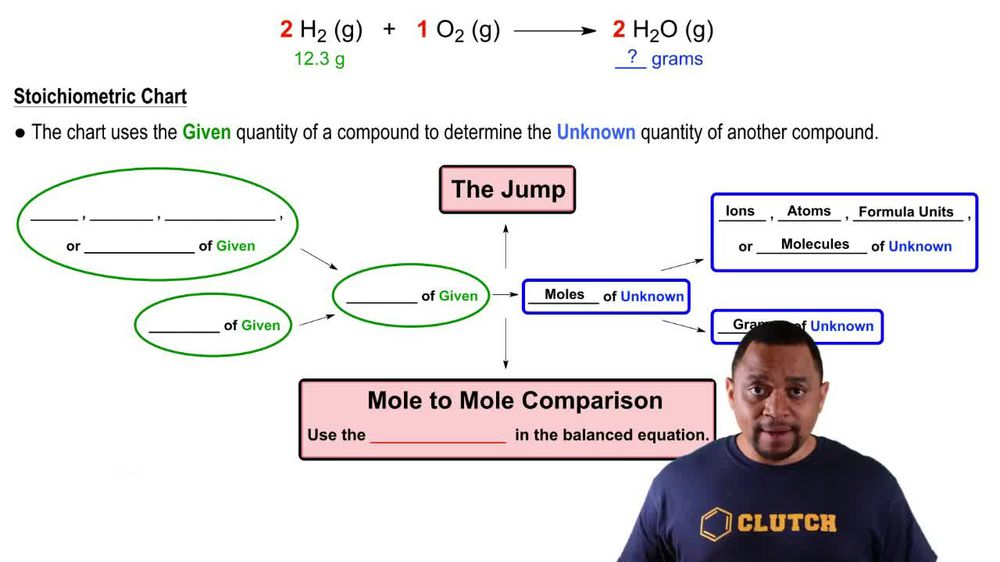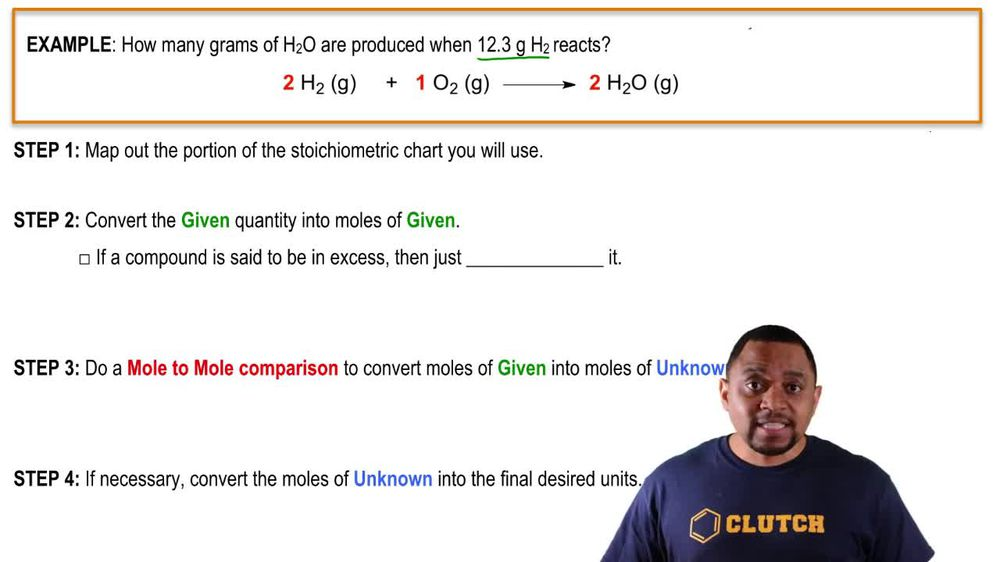Start typing, then use the up and down arrows to select an option from the list.
1. 3. Chemical Reactions2. Stoichiometry# Stoichiometry

by Jules Bruno
381 views
2
2
when it comes to chemical equations, it becomes important to first balance your chemical equation because this will later on lead us to our understanding of story geometry. Now, story geometry deals with the numerical relationship between compounds in a balanced chemical equation. It allows us to determine the amount of products from reactant and vice versa. For example, here we have our chemical equation. That's balance we have to Hydrogen gas is reacting with one oxygen gas to produce to water molecules as gas. They're giving us 12.3 g of H two, and they're asking us to determine the grams of H 20 H. 20 gas produced. This is what store geometry is. They're giving us a balanced chemical equation. They're giving us information on one of the compounds within this balanced equation and asking us to find another compound within that same balanced equation. Now that we know what's dark geometry is, how exactly do we do it? Well, if you click on the next video, we'll take a look at the procedures that you'll need to employ in order to do any type of stoke geometric calculation.